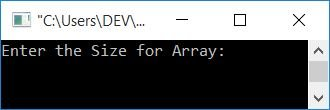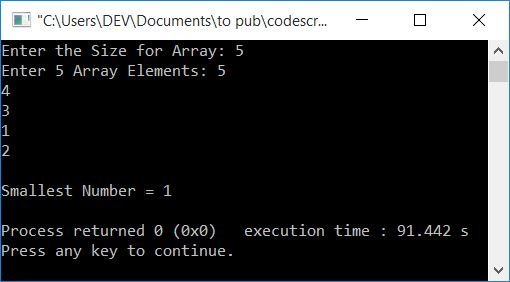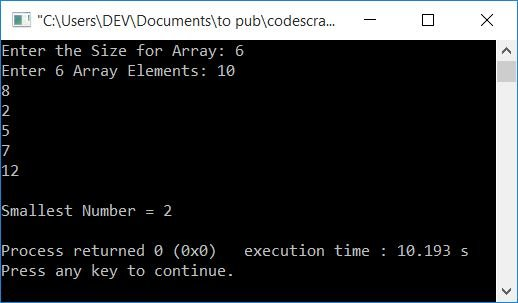# C++ Program to Find the Smallest Number in an Array

In this article, you will learn and get code to find and print the smallest element (number) from an array in C++. The size and elements of the array must be entered by the user at run-time. Here is the list of approaches used to create the program:

## Find the smallest number in an array

To find the smallest element or number in an array in C++ programming, you have to ask the user to enter the size and elements of the array. Now find and print the smallest one as shown in the program given below:

The question is, "Write a program in C++ to find and print the smallest number in an array." Here is its answer:

```#include<iostream>
using namespace std;
int main()
{
int arr, tot, i, s;
cout<<"Enter the Size for Array: ";
cin>>tot;
cout<<"Enter "<<tot<<" Array Elements: ";
for(i=0; i<tot; i++)
cin>>arr[i];
s = arr;
for(i=1; i<tot; i++)
{
if(s>arr[i])
s = arr[i];
}
cout<<"\nSmallest Number = "<<s;
cout<<endl;
return 0;
}```

This program was built and runs under the Code::Blocks IDE. Here is its sample run:Now supply any number, say 5, as the array size, and then enter 5 numbers as the 5 array elements. After supplying all these things, press the ENTER key to find and print the smallest number from the array, as shown in the snapshot given below:The following statement:

`s = arr;`

It is supposed that the number at the 0th (very first) index is the smallest number. And then we've compared all the numbers at the remaining indexes one by one with the number at s. If s's value is found to be greater than any number at any index, then we've initialized that number as the new value of s.

The dry run of the above program goes like this:

• When the user enters the size, say 5, for the array, then it gets stored in tot. So tot=6
• Now we've created a for loop that executes six times. That is, the loop variable, i, starts from 0 to 5.
• So at first, the first number gets stored in arr, the second number in arr, and so on.
• Therefore, if the user enters 5 numbers as 5, 4, 3, 1, 2, then it gets stored in arr[] in this way:
• arr=5
• arr=4
• arr=3
• arr=1
• arr=2
• Now consider the statement that assumes the first number is the smallest number. In other words, arr or 5 is initialized to s.
• Now, using the second for loop, we've compared the value of s with each and every element of arr.
• That is, at first execution of the forloop , 1 gets initialized to i, and the condition i<tot or 1<5 evaluates to be true, therefore program flow goes inside the loop, and the condition s>arr[i] or 5>arr or 5>4 evaluates to be true, therefore program flow goes inside the if's body, and the value of arr[i] or arr or 4 gets initialized to s.
• Now the program flow goes to the update part of the for loop and increments the value of i. So i=2
• Now the condition of the for loop gets evaluated again. Before entering the body of the loop, condition must be evaluated to be true each time.
• This time again, the condition i<tot or 2<5 evaluates to be true, therefore the program flow again goes inside the loop.
• This process continues until the condition is evaluated as false.
• After exiting the loop, the variable s holds the smallest number from the given list of numbers stored in arr.
• So just print the value of s as the smallest number from an array entered by the user.

### Using the pointer, find the smallest number in the array

Now, let's make the same program with pointers. The & is known as the address of operator, where * is called as the value at operator.

```#include<iostream>
using namespace std;
int main()
{
int arr, tot, i, s, *ptr;
cout<<"Enter the Size for Array: ";
cin>>tot;
cout<<"Enter "<<tot<<" Array Elements: ";
for(i=0; i<tot; i++)
cin>>arr[i];
ptr = &arr;
s = *ptr;
while(*ptr)
{
if(s>(*ptr))
s = *ptr;
ptr++;
}
cout<<"\nSmallest Number = "<<s;
cout<<endl;
return 0;
}```

This program produces the same output as the previous program. after executing the following statement:

`ptr = &arr;`

The address of the number present at the 0th index in arr[] gets initialized to ptr. The *ptr indicates the value present at address, which is stored in ptr. And the ptr++ is used to shift to the next index of the same array, arr[].

### Find the smallest number in an array using a function

This is the last program on finding the smallest element in an array. This program is created using a user-defined function named findSmallest(). This function takes an array and its size as its arguments. and returns the smallest number from the array (passed as its first argument).

```#include<iostream>
using namespace std;
int findSmallest(int [], int);
int main()
{
int arr, tot, i, s;
cout<<"Enter the Size for Array: ";
cin>>tot;
cout<<"Enter "<<tot<<" Array Elements: ";
for(i=0; i<tot; i++)
cin>>arr[i];
s = findSmallest(arr, tot);
cout<<"\nSmallest Number = "<<s;
cout<<endl;
return 0;
}
int findSmallest(int a[], int t)
{
int s, i;
s = a;
for(i=1; i<t; i++)
{
if(s>a[i])
s = a[i];
}
return s;
}```

Here is its sample run with user input, 6 as array size, and 10, 8, 2, 5, 7, and 12 as 6 array elements:#### The same program in different languages

C++ Quiz

« Previous Program Next Program »